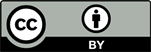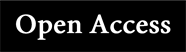Study on the Characteristics of Laser-Induced Plasma in Tieguanyin Tea<sup> </sup>

Jingwen Li

School of Science, Tianjin University of Technology, Tianjin

Received: May 11th, 2023; accepted: Jul. 5th, 2023; published: Jul. 13th, 2023

ABSTRACT

China is the hometown and birthplace of tea. Nowadays, tea culture has spread to all over the world and tea is the second largest beverage after water. In this study, the elemental composition of Tieguanyin tea was studied by laser-induced breakdown spectroscopy (LIBS). Ca, Na, Mg, K, Fe, Cu, Zn, Mn, Sr, Al, Li and Ba were found in Tieguanyin tea. The spectral lines of CN and C2 were also detected. Then, the plasma characteristics of Tieguanyin tea of laser-induction were researched. The electron density (Ne) was 1.44 × 1017 cm−3 by using the Lorentz fitting of the Hα line. The Saha-Boltzmann plot was established, and the plasma temperature (T) was calculated to be 8161 K. Finally, LIFBASE software was used to simulate CN ( Δ ν = 0 ) molecule, and the vibration temperature (Tv) and rotation temperature (Tr) were obtained as 6800 K and 5800 K, respectively. In the experiment, the three temperatures met the relationship T > Tv > Tr, which was consistent with the actual situation of plasma evolution over time.

Keywords:Laser-Induced Breakdown Spectroscopy, Tea, CN Free Radical, Plasma Temperature, Electron Density1. 引言

2. LIBS实验装置

3. 结果与讨论3.1. 铁观音茶叶的LIBS光谱图

3.2. 等离子体特性的研究

1) 等离子体中各元素含量与样品中各元素含量相同；

2) 局域热力学平衡；

3) 光学薄(主要电离过程来自于热电子的碰撞激发过程)。

I λ k i = A k i n k s = n s A k i g k e − E k k T U s ( T ) . (1)

I ¯ λ k i = F n s A k i g k e − E k k T U s ( T ) = F n p C s A k i g k e − E k k T U s ( T ) . (2)

y = ln I ¯ λ k i A k i g k , x = E k , m = − 1 k T , q s = ln F n p C s U s ( T ) . (3)

y = m x + q s . (4)

C s = U s ( T ) e q s F n p . (5)

∑ s ′ C s ′ = 1 F n p ∑ s ′ U s ′ ( T ) q s ′ = 1. (6)

F n p = ∑ s ′ U s ′ ( T ) q s ′ . (7)

C s = U s ( T ) e q s ∑ s ′ U s ′ ( T ) q s ′ . (8)

C s a C s b = U s a ( T ) e q s a U s b ( T ) e q s b . (9)

n I I n I = ( 2 π m e k T ) 3 / 2 n e h 3 2 U I I ( T ) U I ( T ) e − E ion k T . (10)

I j h I I = n I I A j h I I g j I I e − E j I I k T U I I ( T ) = A j h I I g j I I e − E j I I k T U I ( T ) n I n e 2 ( 2 π m e ) 3 / 2 h 3 ( k T ) 3 / 2 e − E ion k T . (11)

ln ( I ¯ j h I I A j h I I g j I I ) = ln ( F n p C I U I ( T ) ) + ln ( 2 ( 2 π m e k T ) 3 / 2 n e h 3 ) − ( E ion + E j I I k T ) . (12)

x * = { E k 原 子 线 E j + E ion 离 子 线 (13)

y * = { ln I ¯ λ k i A k i g k 原 子 线 ln ( I ¯ j h I I A j h I I g j I I ) − ln ( 2 ( 2 π m e k T ) 3 / 2 n e h 3 ) 离 子 线 (14)

Δ E = z e 2 4 π ε 0 ρ ′ D , (15)

ε 0 (F m−1)为真空中介电常数， ρ ′ D (nm)为德拜半径。德拜半径

ρ ′ D = ε 0 k T e 2 ( n e + ∑ z z 2 n z ) , (16)

Δ E = z e 3 4 π n e ε 0 3 k T . (17)

n e ( cm − 3 ) = 10 17 ( FWHA ( nm ) / 0.549 ) 1.4713 (19)

3.3. CN分子的振动温度和转动温度

4. 结论